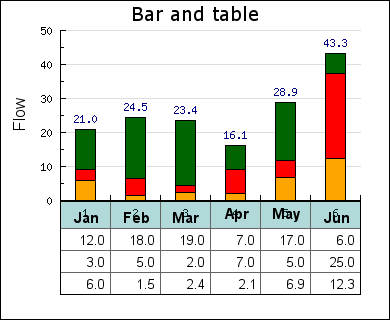## 19.6. Case study: Adding a table to a bar graph

The goal of this example is to explain in detail how to achieve the graphs shown in Figure 19.20

Figure 19.20. Combining a bar graph and a table `(tablebarex1.php)`The graph in Figure 19.20 consists of a standard accumulated bar graph combined with a table just below the X-axis. The way to create such a graph is very basic. First create a standard bar graph with some extra bottom margin (to make room for the table) and then create and position the table below the graph. The only challenge here is to make sure that the width of the table and graph matches to line up each column under each bar.

Lets walk through the steps to do this.

The data to be plotted in the bar and in the table is created in a table as follows

 ```1 2 3 4 5 6 7 ``` ``````

each line in this matrix corresponds to one row in the table and one set of bars that will make up the accumulated bar plot.

First let's define some constants that will control the size and position of the graph and table.

 ```1 2 3 4 5 6 7 8 9 ``` ``````

The next step is to calculate a suitable size for the entire graph, i.e. the entire image.

 ```1 2 3 4 ``` ``````

Finally its time ot make use of these constants to rceate the basic graph. Note that we calculate the bottom margin to just fit the tables selected Y-coordinate position.

 ```1 2 3 4 5 6 ``` ```img->SetMargin(\$tablexpos,\$rightmargin,\$topmargin,\$height-\$tableypos); \$graph->SetScale('textlin'); \$graph->SetMarginColor('white'); ?>```

The rest of the code to create the accumulated bar plot is pretty mundane and we just show the code here without further comment.

 ```1 2 3 4 5 6 7 8 9 10 11 12 ``` ```SetFillColor('orange'); \$bplot2 = new BarPlot(\$datay); \$bplot2->SetFillColor('red'); \$bplot3 = new BarPlot(\$datay); \$bplot3->SetFillColor('darkgreen'); \$accbplot = new AccBarPlot(array(\$bplot,\$bplot2,\$bplot3)); \$accbplot->value->Show(); \$graph->Add(\$accbplot); ?>```

It's now time to setup the table. The position of the table is determined by the previous set constants and the original data as specified above.

 ```1 2 3 4 5 ``` ```Set(\$datay); \$table->SetPos(\$tablexpos,\$tableypos+1); ?>```

The rest of the table formatting is fairly basic and again we just show the necessary script without further comment.

 ```1 2 3 4 5 6 7 8 9 10 11 12 13 14 ``` ```SetFont(FF_ARIAL,FS_NORMAL,10); \$table->SetAlign('right'); \$table->SetMinColWidth(\$cellwidth); \$table->SetNumberFormat('%0.1f'); // Format table header row \$table->SetRowFillColor(0,'teal@0.7'); \$table->SetRowFont(0,FF_ARIAL,FS_BOLD,11); \$table->SetRowAlign(0,'center'); // .. and add it to the graph \$graph->Add(\$table); ?>```